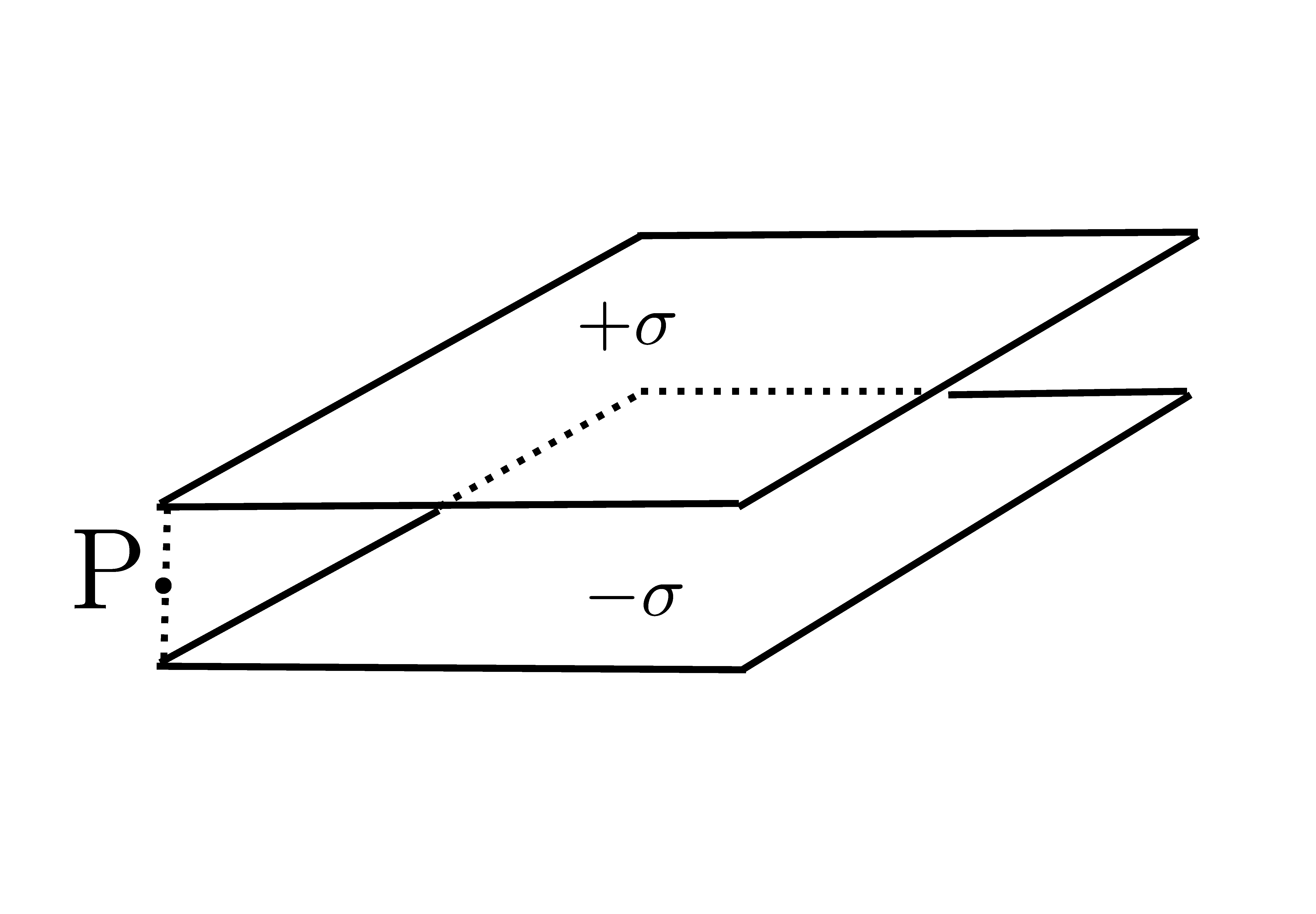# Edge effects in the parallel plate capacitor.

As we know, the simplest capacitor, consists of two conductive plates separated by air (or any other dielectric). If the surface charge density of the plates are $\pm \sigma$ then we know that there is a homogeneous electric field between the plates given by $E=\frac{\sigma}{\epsilon_{0}}$ However, this simple expression for the electric field only works when the dimensions of the plates are much greater than its separation. In addition, the above formula only describes the field correctly at points which are far from the edges of the capacitor. Far from the center of the capacitor, the so-called "edge effects" play an important role and the magnitude of the electric field deviates from $\frac{\sigma}{\epsilon_{0}}$. In order to verify this, determine the magnitude of the electric field in volts per meter at a point P located at the corner of the capacitor and right between the plates. The surface charge density of the plates is $\sigma= 1~\mbox{nC/m}^{2}$.Details and assumptions

$\epsilon_{0}=8.85\times 10^{-12}~\mbox{F}\cdot \mbox{m}^{-1}$

×